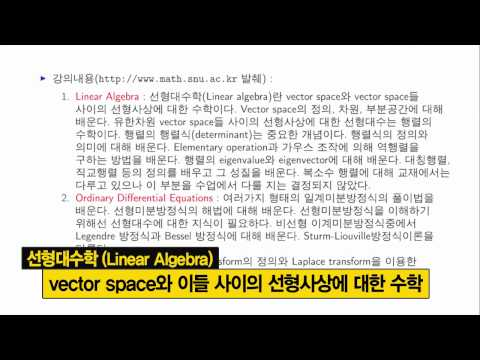## 과정 안내

SNUON의 다양한 강좌들을 수강신청 후 수강하실 수 있습니다.### 공학수학 1 (SHB)

모집기간 : 2020-09-01 ~ 2021-02-28
학습기간 : 2020-09-01 ~ 2021-02-28
• 강좌 정보 및 소개

학습목차

1. Introduction to matrix

2. System of linear equations, Gauss elimination

3. Rank of a matrix, Linear independence of vectors

4. Vector space

5. Determinant of a matrix

6. Inverse of a matrix

7. Eigenvalues and eigenvectors

8. Similarity transformation, diagonalization, and quadratic form

9. Introduction to differential equation

10. Solving first order differential equations

11. More on first order differential equations

12. Solving the second order linear DE

13. The second order linear DE

14. Second order nonhomogeneous linear DE

15. Higher order linear DE

16. Case studies

17. Systems of ODEs

18. Qualitative properties of systems of ODE

19. Linearization and nonhomogeneous linear systems of ODE

20. Series solutions of ODE

21. Frobenius method

22. Bessel DE and Bessel functions

23. Laplace transform Ⅰ

24. Laplace transform Ⅱ

25. Laplace transform Ⅲ

• 강좌코드 : 2020_80_C_SHB_04_006_2020_2
• 과정 : 공학수학 1
• 주수 : 25
• 수강가능수 : 100000
• 학점 : 0
• 언어 : 한국어 (ko)
• 태그 : 공학수학, 공학수학1, 심형보, 학내공개
이전페이지